## » Noise and Ray Marching

» September 26, 2021 | development english math | Adrian Kummerländer

LiterateLB's volumetric visualization functionality relies on a simple ray marching implementation to sample both the 3D textures produced by the simulation side of things and the signed distance functions that describe the obstacle geometry. While this produces surprisingly nice looking results in many cases, some artifacts of the visualization algorithm are visible depending on the viewport and sample values. Extending the ray marching code to utilize a noise function is one possibility of mitigating such issues that I want to explore in this article.

While my original foray into just in time visualization of Lattice Boltzmann based simulations was only an aftertought to playing around with SymPy based code generation approaches I have since put some work into a more fully fledged code. The resulting LiterateLB code combines symbolic generation of optimized CUDA kernels and functionality for just in time fluid flow visualization into a single literate document.

For all fortunate users of the Nix package manager, tangling and building this from the Org document is as easy as executing the following commands on a CUDA-enabled NixOS host.

git clone https://code.kummerlaender.eu/LiterateLB
nix-build
./result/bin/nozzle


### Image Synthesis

The basic ingredient for producing volumetric images from CFD simulation data is to compute some scalar field of samples $s : \mathbb{R}^3 \to \mathbb{R}_0^+$. Each sample $s(x)$ can be assigned a color $c(x)$ by some convenient color palette mapping scalar values to a tuple of red, green and blue components.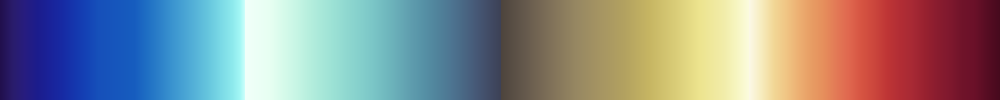The task of producing an image then consists to sampling the color field along a ray assigned to a pixel by e.g. a simple pinhole camera projection. For this purpose a simple discrete approximation of the volume rendering equation with constant step size $\Delta x \in \mathbb{R}^+$ already produces suprisingly good pictures. Specifically

$C(r) = \sum_{i=0}^N c(i \Delta x) \mu (i \Delta x) \prod_{j=0}^{i-1} \left(1 - \mu(j\Delta x)\right)$

is the color along ray $r$ of length $N\Delta x$ with local absorption values $\mu(x)$. This local absorption value may be chosen seperately of the sampling function adding an additional tweaking point.

The basic approach may also be extended arbitrarily, e.g. it is only the inclusion of a couple of phase functions away from being able recover the color produced by light travelling through the participating media that is our atmosphere.

### The Problem

There are many different possibilities for the choice of sampling function $s(x)$ given the results of a fluid flow simulation. E.g. velocity and curl norms, the scalar product of ray direction and shear layer normals or vortex identifiers such as the Q criterion

$Q = \|\Omega\|^2 - \|S\|^2 > 0 \text{ commonly thresholded to recover isosurfaces}$

that contrasts the local vorticity and strain rate norms. The strain rate tensor $S$ is easily recovered from the non-equilibrium populations $f^\text{neq}$ of the simulation lattice — and is in fact already used for the turbulence model. Similarly, the vorticity $\Omega = \nabla \times u$ can be computed from the velocity field using a finite difference stencil.

The problem w.r.t. rendering when thresholding sampling values to highlight structures in the flow becomes apparent in the following picture:

Q Criterion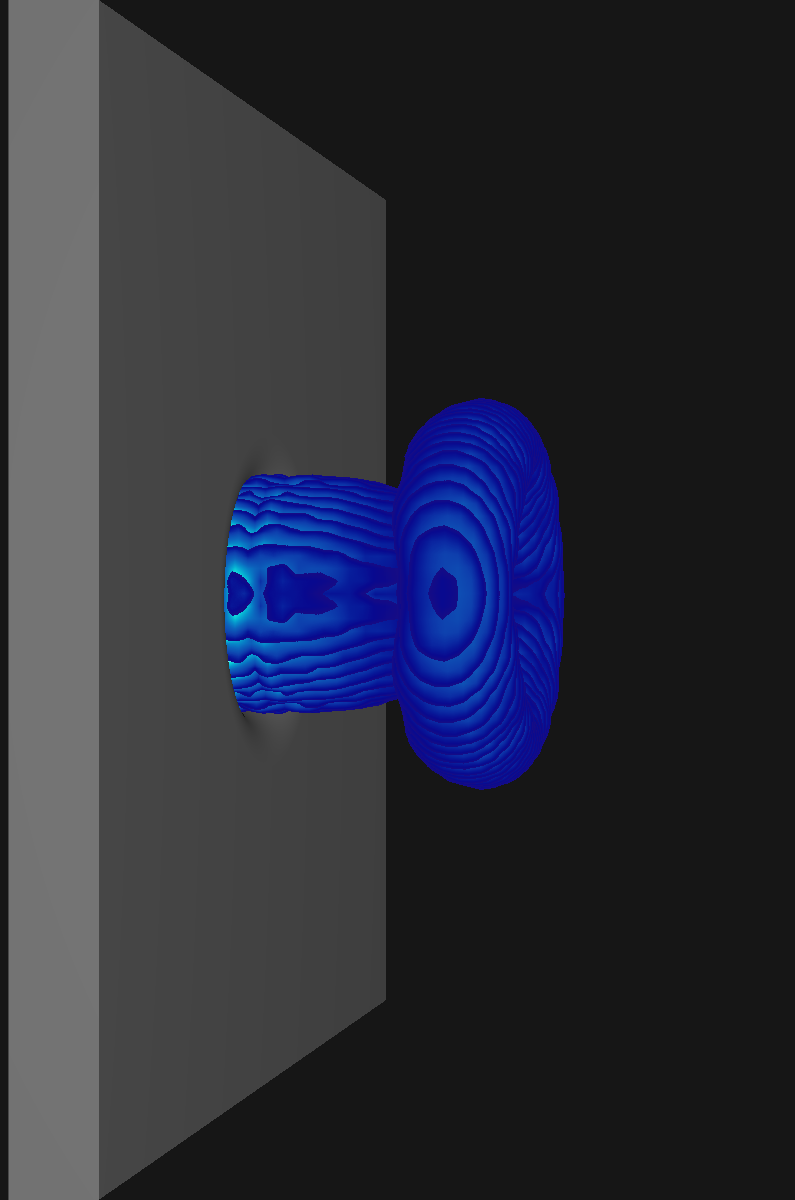Curl Norm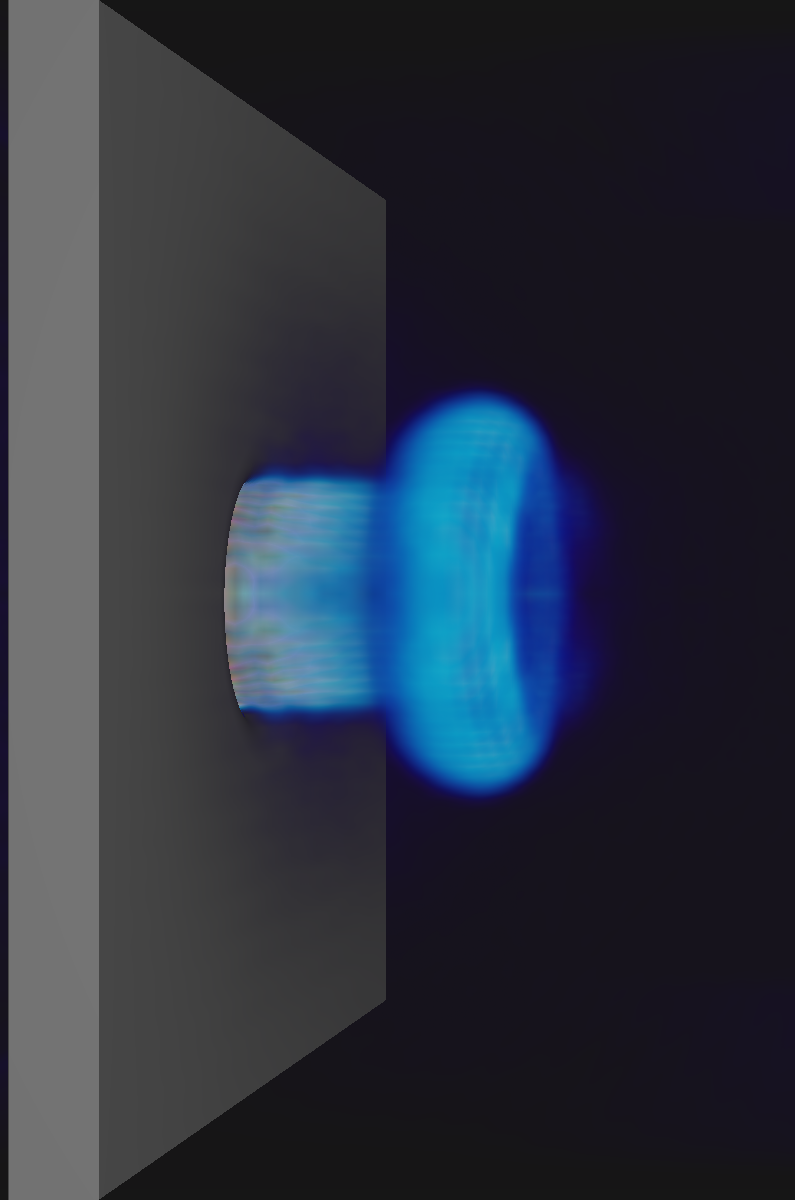While the exact same volume discretization was used for both visualizations, the slices are much less apparent for the curl norm samples due to the more gradual changes. In general the issue is most prominent for scalar fields with large gradients (specifically the sudden jumps that occur when restricting sampling to certain value ranges as is the case for the Q criterion).

### Colors of Noise

The reason for these artifacts is primarily choice of start offsets w.r.t. the traversed volume in addition the the step width. While this tends to become less noticable when decreasing said steps, this is not desirable from a performance perspective.

What I settled on for LiterateLB's renderer are view-aligned slicing and random jittering to remove most visible artifacts. The choice of randomness for jittering the ray origin is critical here as plain random numbers tend to produce a distracting static-like pattern. A common choice in practice is to use so called blue noise instead. While both kinds of noise eliminate most slicing artifacts, the remaining patterns tend to be less noticeable for blue noise. Noise is called blue if it contains only higher frequency components which makes it harder for the pattern recognizer that we call brain to find patterns where there should be none.

The void-and-cluster algorithm1 provides a straight forward method for pre-computing tileable blue noise textures that can be reused during the actual visualization. Tileability is a desirable property for this as we otherwise would either need a noise texture large enough to cover the entire image or instead observe jumps at the boundary between the tiled texture.

The first ingredient for void-and-cluster is a filteredPattern function that applies a plain Gaussian filter with given $\sigma$ to a cyclic 2d array. Using cyclic wrapping during the application of this filter is what renders the generated texture tileable.

def filteredPattern(pattern, sigma):
return gaussian_filter(pattern.astype(float), sigma=sigma, mode='wrap',
truncate=np.max(pattern.shape))


This function will be used to compute the locations of the largest void and tightest cluster in a binary pattern (i.e. a 2D array of 0s and 1s). In this context a void describes an area with only zeros and a cluster describes an area with only ones.

def largestVoidIndex(pattern, sigma):


These two functions work by considering the given binary pattern as a float array that is blurred by the Gaussian filter. The blurred pattern gives an implicit ordering of the voidness of each pixel, the minimum of which we can determine by a simple search. It is important to exclude the initial binary pattern here as void-and-cluster depends on finding the largest areas where no pixel is set.

def tightestClusterIndex(pattern, sigma):
logical_not(pattern)))


Computing the tightest cluster works in the same way with the exception of searching the largest array element and masking by the inverted pattern.

def initialPattern(shape, n_start, sigma):
initial_pattern = np.zeros(shape, dtype=np.bool)
initial_pattern.flat[0:n_start] = True
initial_pattern.flat = np.random.permutation(initial_pattern.flat)
cluster_idx, void_idx = -2, -1
while cluster_idx != void_idx:
cluster_idx = tightestClusterIndex(initial_pattern, sigma)
initial_pattern.flat[cluster_idx] = False
void_idx = largestVoidIndex(initial_pattern, sigma)
initial_pattern.flat[void_idx] = True
return initial_pattern


For the initial binary pattern we set n_start random locations to one and then repeatedly break up the largest void by setting its center to one. This is also done for the tightest cluster by setting its center to zero. We do this until the locations of the tightest cluster and largest void overlap.

def blueNoise(shape, sigma):


The actual algorithm utilizes these three helper functions in four steps:

1. Initial pattern generation

n = np.prod(shape)
n_start = int(n / 10)

initial_pattern = initialPattern(shape, n_start, sigma)
noise = np.zeros(shape)

2. Eliminiation of n_start tightest clusters

pattern = np.copy(initial_pattern)
for rank in range(n_start,-1,-1):
cluster_idx = tightestClusterIndex(pattern, sigma)
pattern.flat[cluster_idx] = False
noise.flat[cluster_idx] = rank

3. Elimination of n/2-n_start largest voids

pattern = np.copy(initial_pattern)
for rank in range(n_start,int((n+1)/2)):
void_idx = largestVoidIndex(pattern, sigma)
pattern.flat[void_idx] = True
noise.flat[void_idx] = rank

4. Elimination of n-n/2 tightest clusters of the inverted pattern

for rank in range(int((n+1)/2),n):
cluster_idx = tightestClusterIndex(np.logical_not(pattern), sigma)
pattern.flat[cluster_idx] = True
noise.flat[cluster_idx] = rank


For each elimination the current rank is stored in the noise texture producing a 2D arrangement of the integers from 0 to n. As the last step the array is divided by n-1 to yield a grayscale texture with values in $[0,1]$.

return noise / (n-1)


In order to check whether this actually generated blue noise, we can take a look at the Fourier transformation for an exemplary $100 \times 100$ texture:

Blue noise texture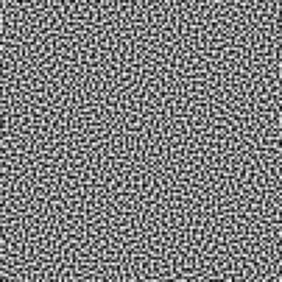Fourier transformation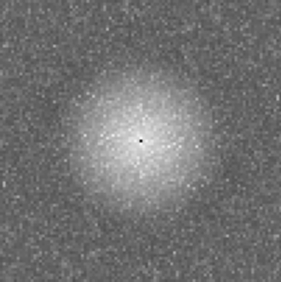One can see qualitatively that higher frequency components are significantly more prominent than lower ones. Contrasting this to white noise generated using uniformly distributed random numbers, no preference for any range of frequencies can be observed:

White noise texture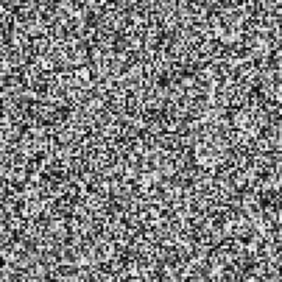Fourier transformation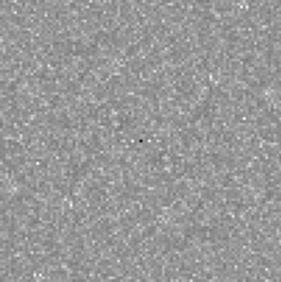### Comparison

Contasting the original Q criterion visualization with one produced using blue noise jittering followed by a soft blurring shader, we can see that the slicing artifacts largely vanish. While the jittering is still visible to closer inspection, the result is significantly more pleasing to the eye and arguably more faithful to the underlying scalar field.

Simple ray marchingRay marching with blue noise jittering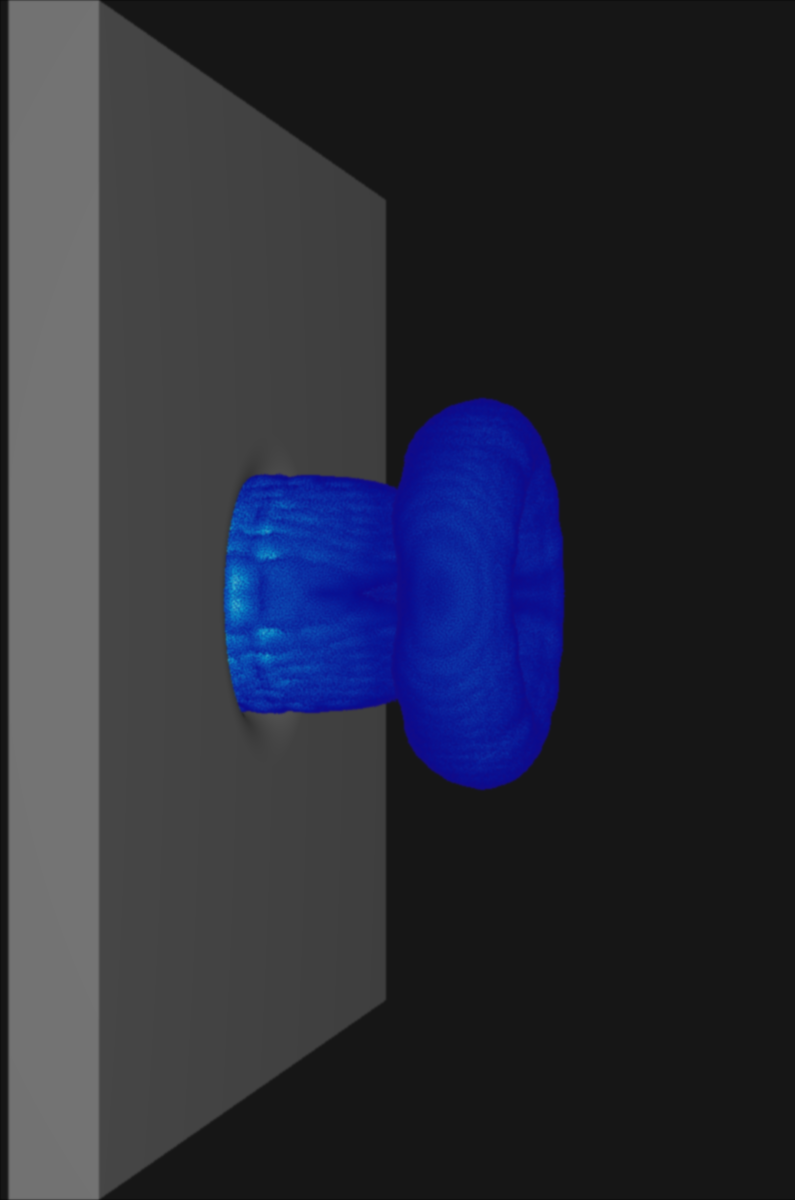While white noise also obcures the slices, its lower frequency components produce more obvious static in the resulting image compared to blue noise. As both kinds of noise are precomputed we can freely choose the kind of noise that will produce the best results for our sampling data.

Blue noise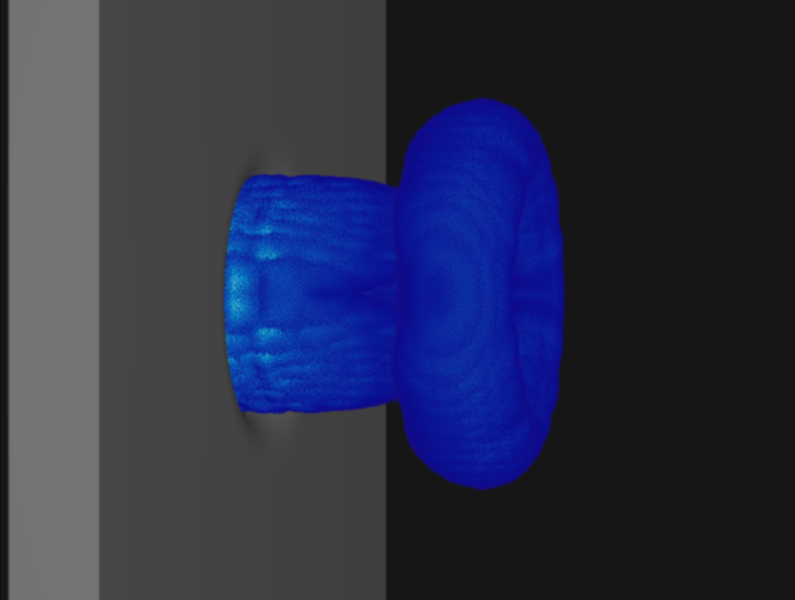White noise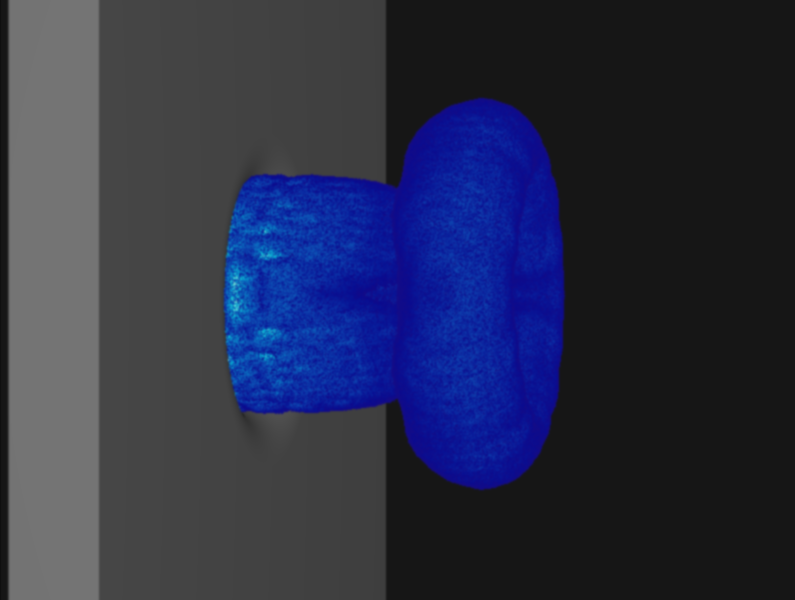In practice where the noise is applied just-in-time during the visualization of a CFD simulation, all remaining artifacts tend to become invisible. This can be seen in the following video of the Q criterion evaluated for a simulated nozzle flow in LiterateLB:

1. Ulichney, R. Void-and-cluster method for dither array generation. In Electronic Imaging (1993). DOI: 10.1117/12.152707.↩︎